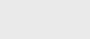2年前 (2021-08-26) |   抢沙发  271

lambda表达式是在Java8中首次引入的。它的主要目标是提高语言的表达能力。

## 什么是Functional Interface？

``````import java.lang.FunctionalInterface;
@FunctionalInterface
public interface MyInterface{
// the single abstract method
double getValue();
}``````

``````public class FunctionInterfaceTest {
public static void main(String[] args) {

// anonymous class
@Override
public void run() {
System.out.println("I just implemented the Runnable Functional Interface.");
}
}).start();
}
}``````

``I just implemented the Runnable Functional Interface.``

Java8进一步扩展了SAMs的功能。因为我们知道函数接口只有一个方法，所以在将其作为参数传递时，不需要定义该方法的名称。Lambda表达式允许我们完全做到这一点。

## lambda表达式简介

Lambda表达式本质上是一个匿名或未命名的方法。lambda表达式不会自行执行。相反，它用于实现由函数接口定义的方法。

``(parameter list) -> lambda body``

``````double getPiValue() {
return 3.1415;
}``````

``() -> 3.1415``

## Lambda体的类型

1. 具有单个表达式的主体

``() -> System.out.println("Lambdas are great");``

2. 由代码块组成的主体。

``````() -> {
double pi = 3.1415;
return pi;
};``````

``````import java.lang.FunctionalInterface;

// this is functional interface
@FunctionalInterface
interface MyInterface{

// abstract method
double getPiValue();
}

public class Main {

public static void main( String[] args ) {

// declare a reference to MyInterface
MyInterface ref;

// lambda expression
ref = () -> 3.1415;

System.out.println("Value of Pi = " + ref.getPiValue());
}
}``````

``Value of Pi = 3.1415``

``````// it will throw an error
MyInterface ref = new myInterface();

// it is valid
MyInterface ref;``````

``ref = () -> 3.1415;``

``System.out.println("Value of Pi = " + ref.getPiValue());``

## 带参数的Lambda表达式

``(n) -> (n%2)==0``

``````@FunctionalInterface
interface MyInterface {

// abstract method
String reverse(String n);
}

public class Main {

public static void main( String[] args ) {

// declare a reference to MyInterface
// assign a lambda expression to the reference
MyInterface ref = (str) -> {

String result = "";
for (int i = str.length()-1; i >= 0 ; i--)
result += str.charAt(i);
return result;
};

// call the method of the interface
System.out.println("Lambda reversed = " + ref.reverse("Lambda"));
}

}``````

``Lambda reversed = adbmaL``

## 通用功能接口

``````@FunctionalInterface
interface MyInterface {
String reverseString(String n);
}``````

``````// GenericInterface.java
@FunctionalInterface
interface GenericInterface<T> {

// generic method
T func(T t);
}

// GenericLambda.java
public class Main {

public static void main( String[] args ) {

// declare a reference to GenericInterface
// the GenericInterface operates on String data
// assign a lambda expression to it
GenericInterface<String> reverse = (str) -> {

String result = "";
for (int i = str.length()-1; i >= 0 ; i--)
result += str.charAt(i);
return result;
};

System.out.println("Lambda reversed = " + reverse.func("Lambda"));

// declare another reference to GenericInterface
// the GenericInterface operates on Integer data
// assign a lambda expression to it
GenericInterface<Integer> factorial = (n) -> {

int result = 1;
for (int i = 1; i <= n; i++)
result = i * result;
return result;
};

System.out.println("factorial of 5 = " + factorial.func(5));
}
}``````

``````Lambda reversed = adbmaL
factorial of 5 = 120``````

• `GenericInterface<String>reverse`-创建对接口的引用。该接口现在对字符串类型的数据进行操作。
• `GenericInterface<Integer>factorial`-创建对接口的引用。在本例中，接口对整型数据进行操作。

## Lambda表达式和StreamAPI

``````import java.util.ArrayList;
import java.util.List;

public class StreamMain {

// create an object of list using ArrayList
static List<String> places = new ArrayList<>();

// preparing our data
public static List getPlaces(){

// add places and country to the list

return places;
}

public static void main( String[] args ) {

List<String> myPlaces = getPlaces();
System.out.println("Places from Nepal:");

// Filter places from Nepal
myPlaces.stream()
.filter((p) -> p.startsWith("Nepal"))
.map((p) -> p.toUpperCase())
.sorted()
.forEach((p) -> System.out.println(p));
}

}``````

``````Places from Nepal:
NEPAL, KATHMANDU
NEPAL, POKHARA``````

``````myPlaces.stream()
.filter((p) -> p.startsWith("Nepal"))
.map((p) -> p.toUpperCase())
.sorted()
.forEach((p) -> System.out.println(p));``````### 注册